# Optimal power limit for deep learning tasks on RTX 3090

So I've recently bought a used RTX 3090 for my experiments with training some nets and found out how much power it consumes and how hot it is.
The stock power limit is 390 watts and can be increased up to 480 watts. The GPU generates so much heat that my CPU temperatures noticeably increase. Running the GPU at 80% fan usage with temperatures reaching 70°C is quite hot, so I began researching methods to reduce the heat. The main method for reducing heat is undervolting: less voltage => less power => less heat. Unfortunately linux drivers don't support undervolting. So the only option left is to reduce power limit.
Power limit is the maximum amount of power GPU can draw. This limit is maintained by adjusting frequencies and voltages to ensure that power consumption remains under the specified limit. I reduced the power limit to 250 watts, and the performance didn't drop as much as I had expected. I've decided to explore how power limit affects different dl workloads:

• Training:
• fp32
• tf32
• amp fp16
• fp16 (.half())
• Inference:
• fp32
• tf32
• amp fp16
• fp16 (.half())
• TensorRT fp16

All tests were conducted with vit_base_patch16_224 from timm library.

# fp32 training batch_size=160 approx. 20GB of VRAM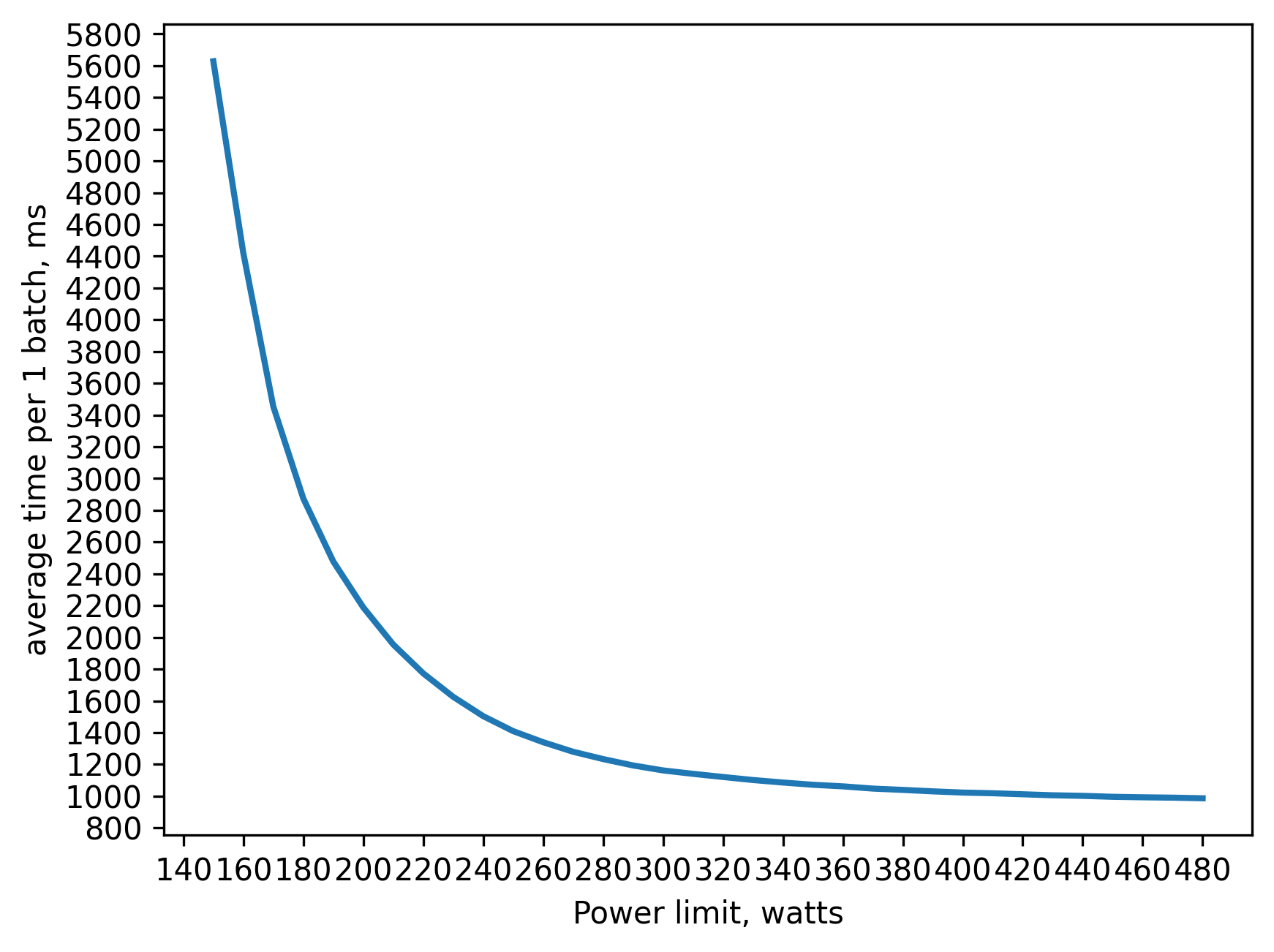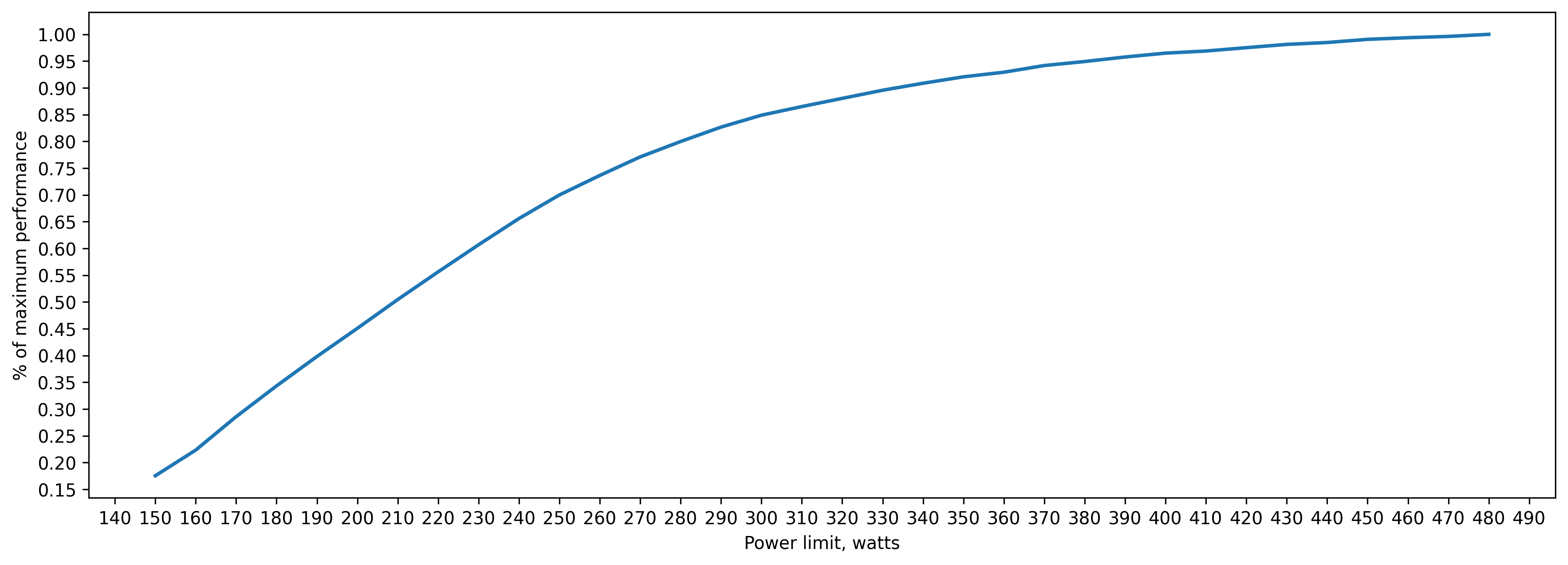280 watts => 80 percent of peak performance(480 watts)
330 watts => 90 percent
380 watts => 95 percent

# tf32

by using

``````torch.backends.cuda.matmul.allow_tf32 = True
``````

we can tell pytorch to use TF32 computation mode. Basically, we can sacrifice some of the fp32 precision to get faster matrix operations. Images below describe how it works and were taken from a blog post by NVIDIA. TF32 operations can be accelerated by Tensor Cores.# tf32 training batch_size=160 approx. 20GB of VRAM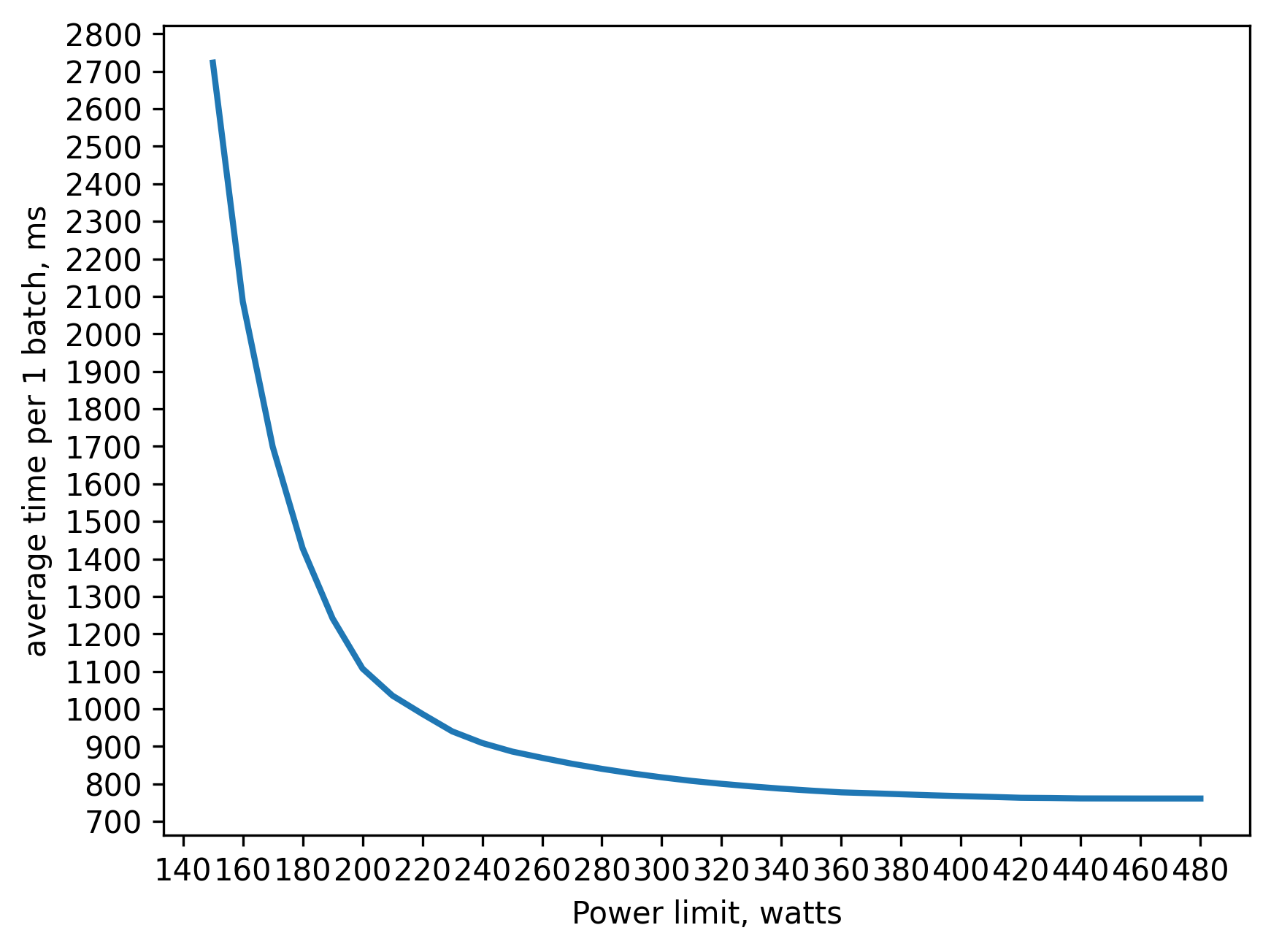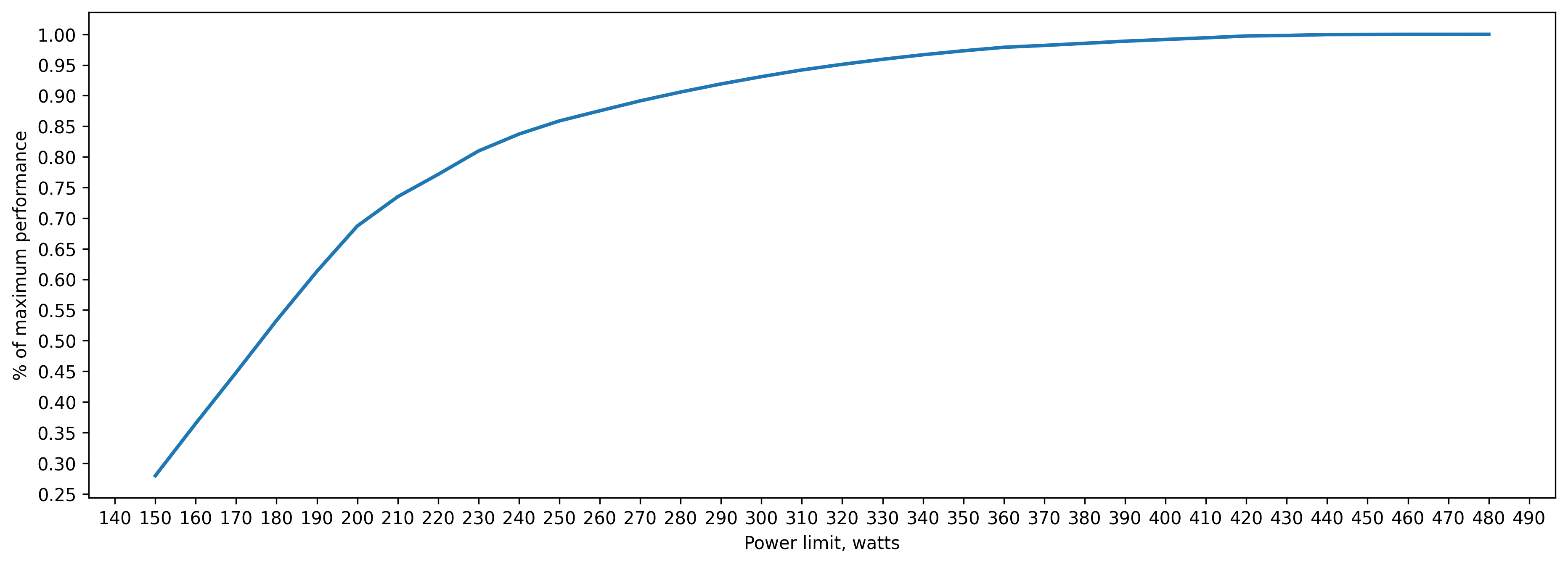230 watts => 80 percent of peak performance(480 watts)
280 watts => 90 percent
320 watts => 95 percent
as we can see, Tensor Cores are not only faster but also much more energy efficient.

# amp (fp16)

by using `torch.autocast` we can tell pytorch to use fp16 instead of fp32 for some operations. List of operations. Once again we trade precision for performance. We can see, that some ops like matmul, conv2d are autocasted to fp16, but ops that require better precision, like sum, pow, loss functions and norms are autocasted to fp32.
There is one problem with using fp16: sometimes gradients are so small, they can't be represented in fp16 and are rounded to zero.
zero gradient => zero weight update => no learning.
This is why we multiply loss values by a scaling factor (which is estimated automatically), so that after backward pass we get non-zero gradient values. Before using gradients for weight updates, we convert them to fp32 and unscale them, so that our scaling doesn't interfere with learning rate.
If certain criteria are met, like cuBLAS/cuDNN version and dimensions of the matrices are right, operations will be carried out by tensor cores. NVIDIA slides 18-19.

``````for images, labels in zip(data, targets):
with autocast(device_type='cuda', dtype=torch.float16):
outputs = model.forward(images)
loss = criterion(outputs,labels)
scaler.scale(loss).backward()
scaler.step(optimizer)
scaler.update()
``````

# amp (fp16) training batch_size=160 approx. 13GB of VRAM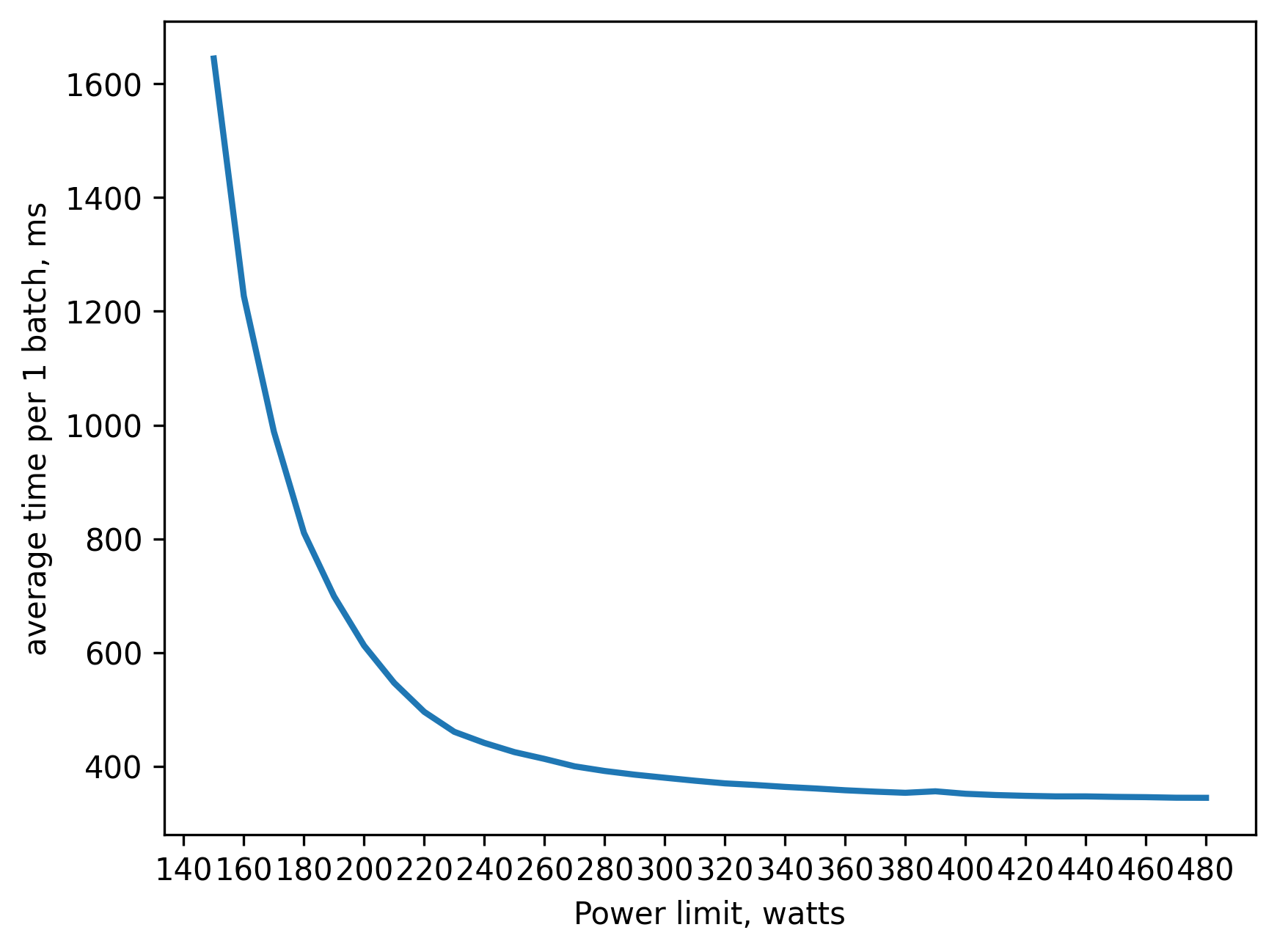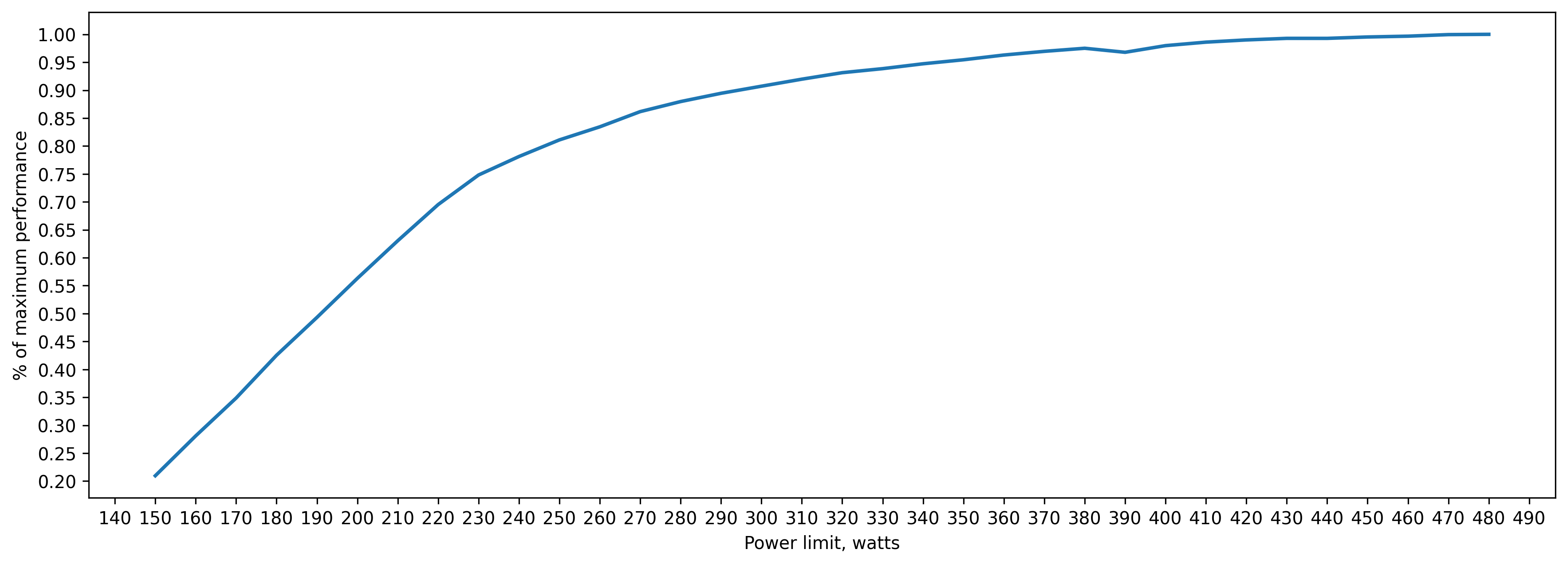250 watt => 80 percent of peak performance(480 watts)
300 watt => 90 percent
350 watt => 95 percent

Power efficiency is a little bit worse than tf32, because some operations are fp32 and don't use Tensor Cores.

# fp16 (.half())

by using `.half()` on our data and model, we convert them to fp16. This is faster than amp but leads to unstable training and can result in a lot of NaNs.

# fp16 (.half()) training batch_size=160 approx. 11GB of VRAM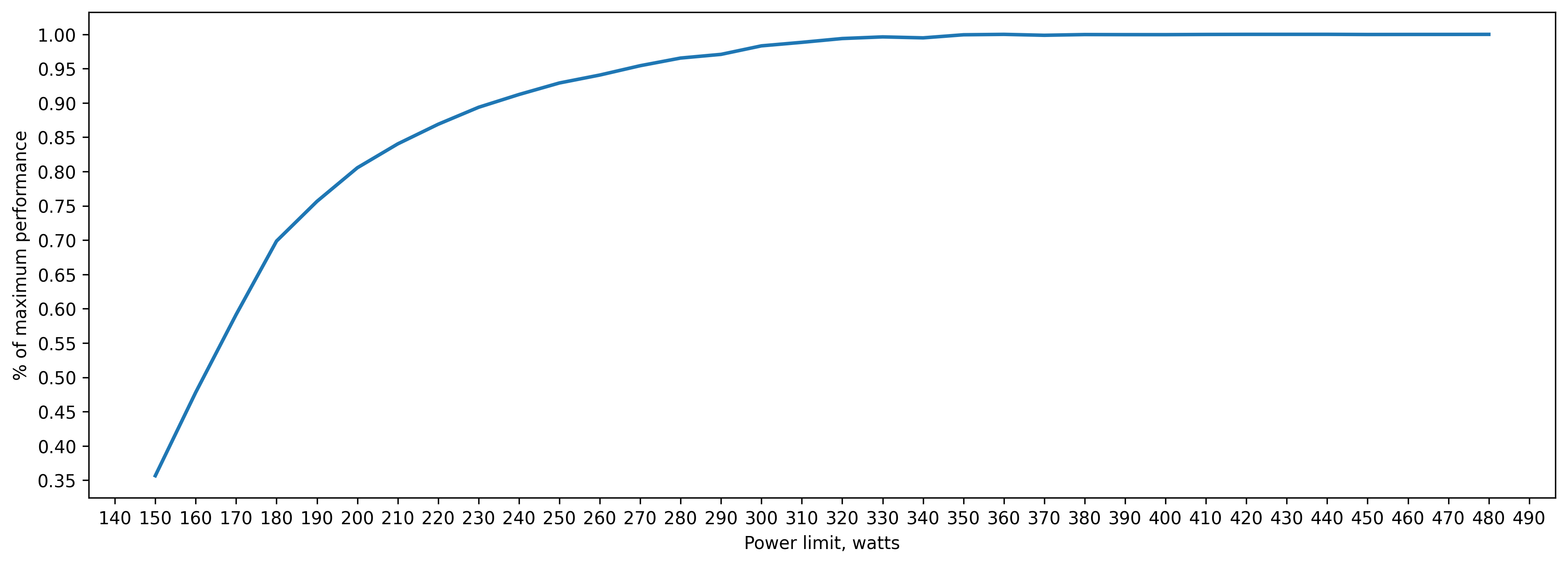200 watt => 80 percent of peak performance(480 watts)
230 watt => 90 percent
270 watt => 95 percent

This is the most power efficient solution: we don't use fp32 at all.

# fp32 inference batch_size=2048 approx. 21GB of VRAM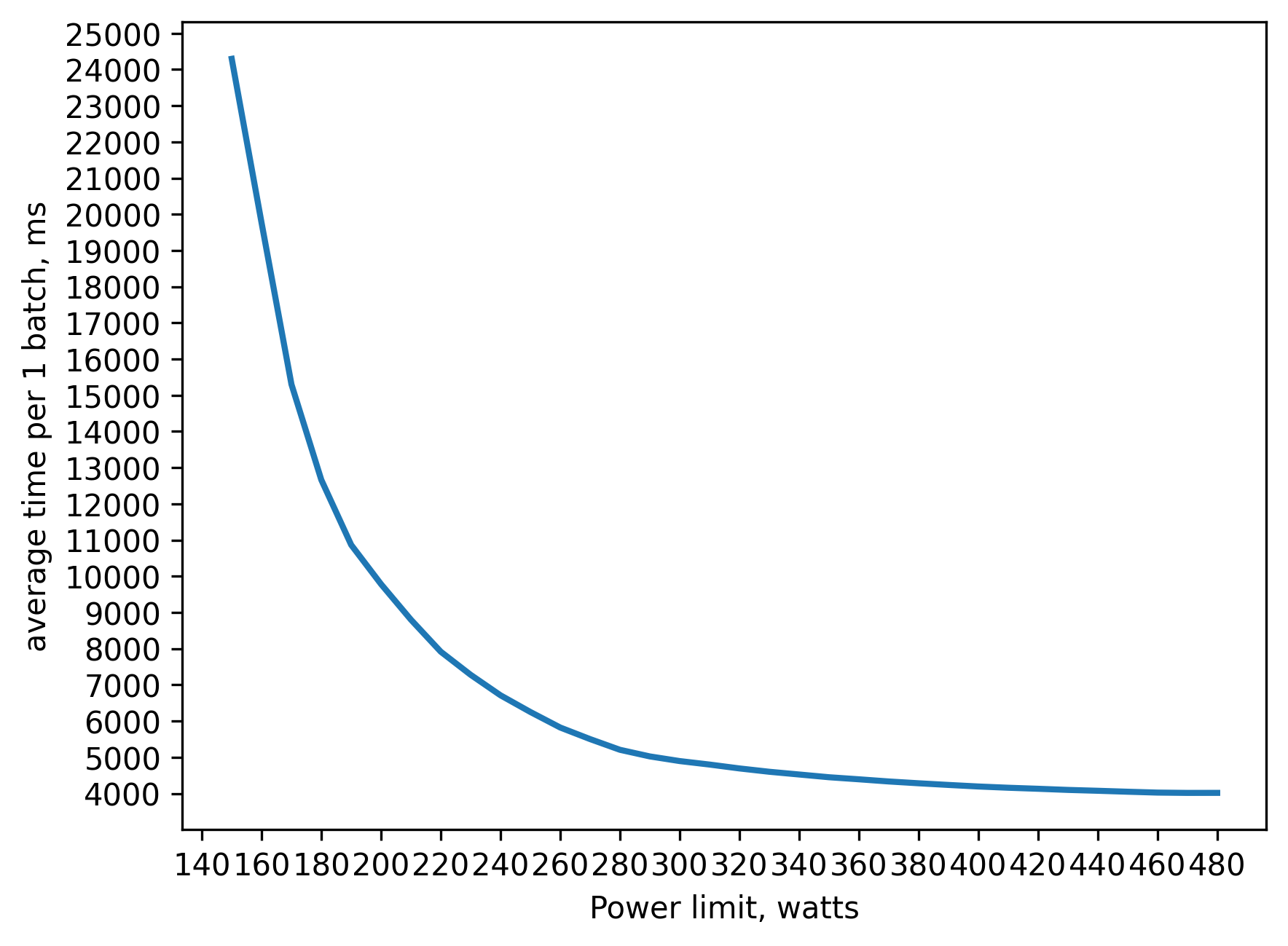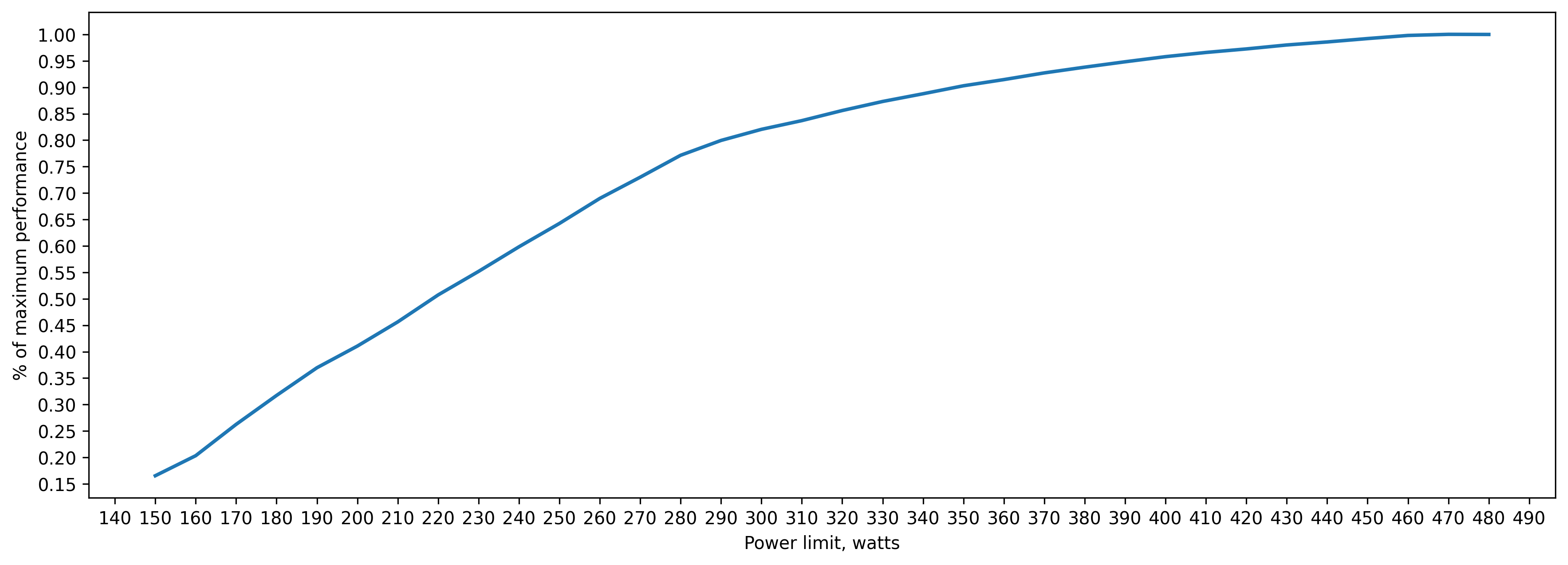290 watt => 80 percent of peak performance(480 watts)
350 watt => 90 percent
390 watt => 95 percent

# tf32 inference batch_size=2048 approx. 21GB of VRAM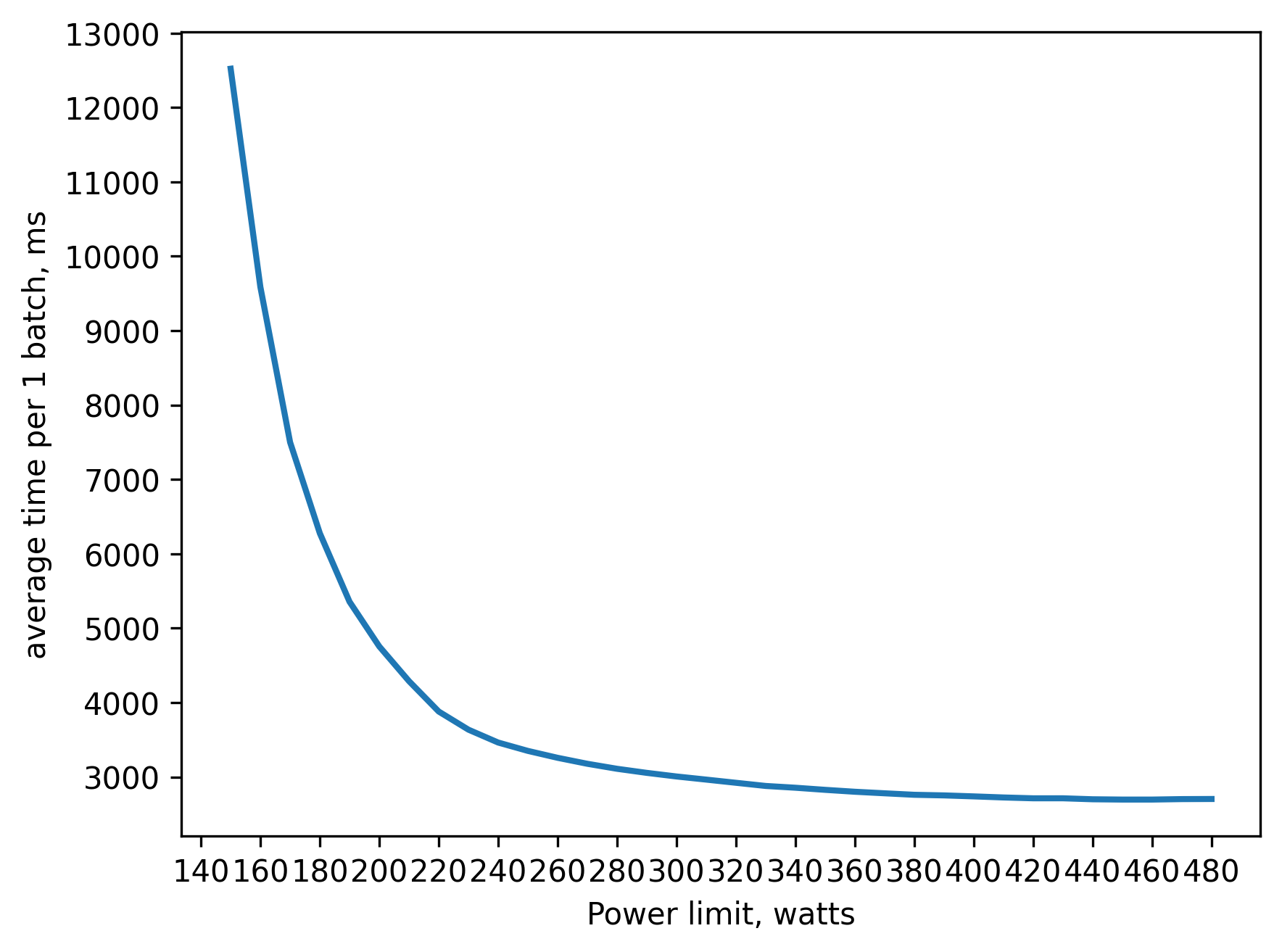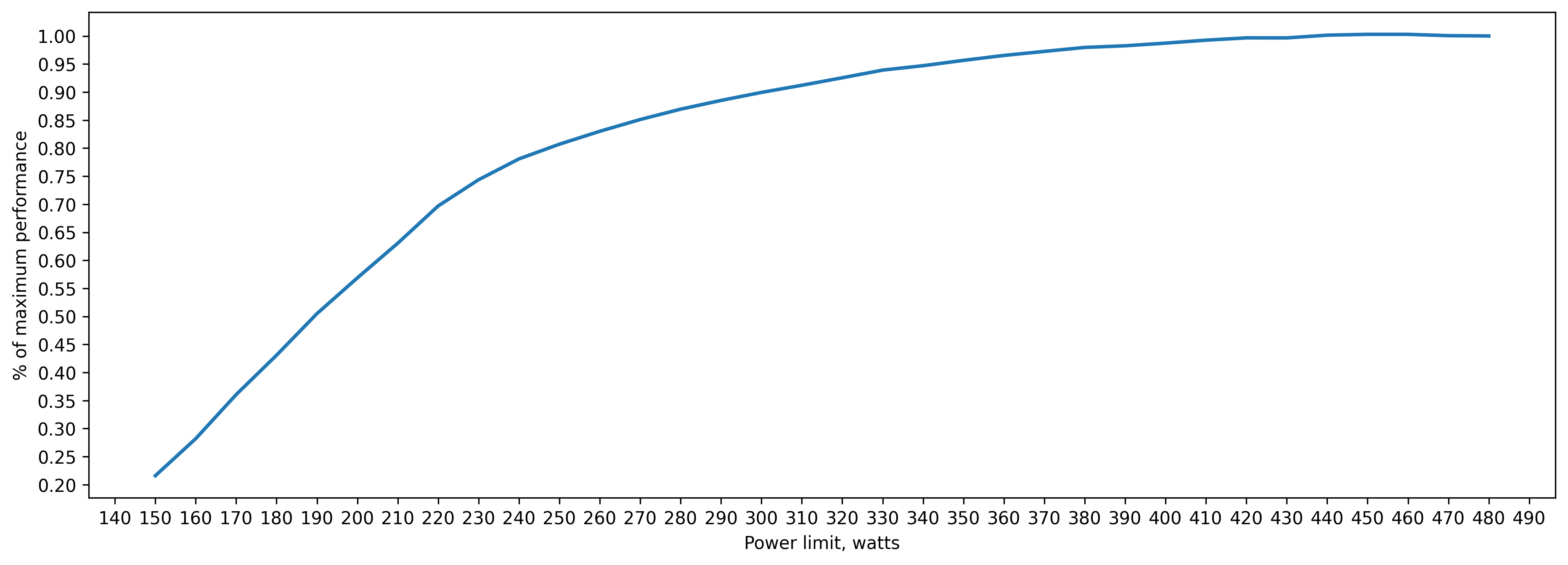250 watts => 80 percent of peak performance(480 watts)
300 watts => 90 percent
350 watts => 95 percent

# amp (fp16) inference batch_size=2048 approx. 16GB of VRAM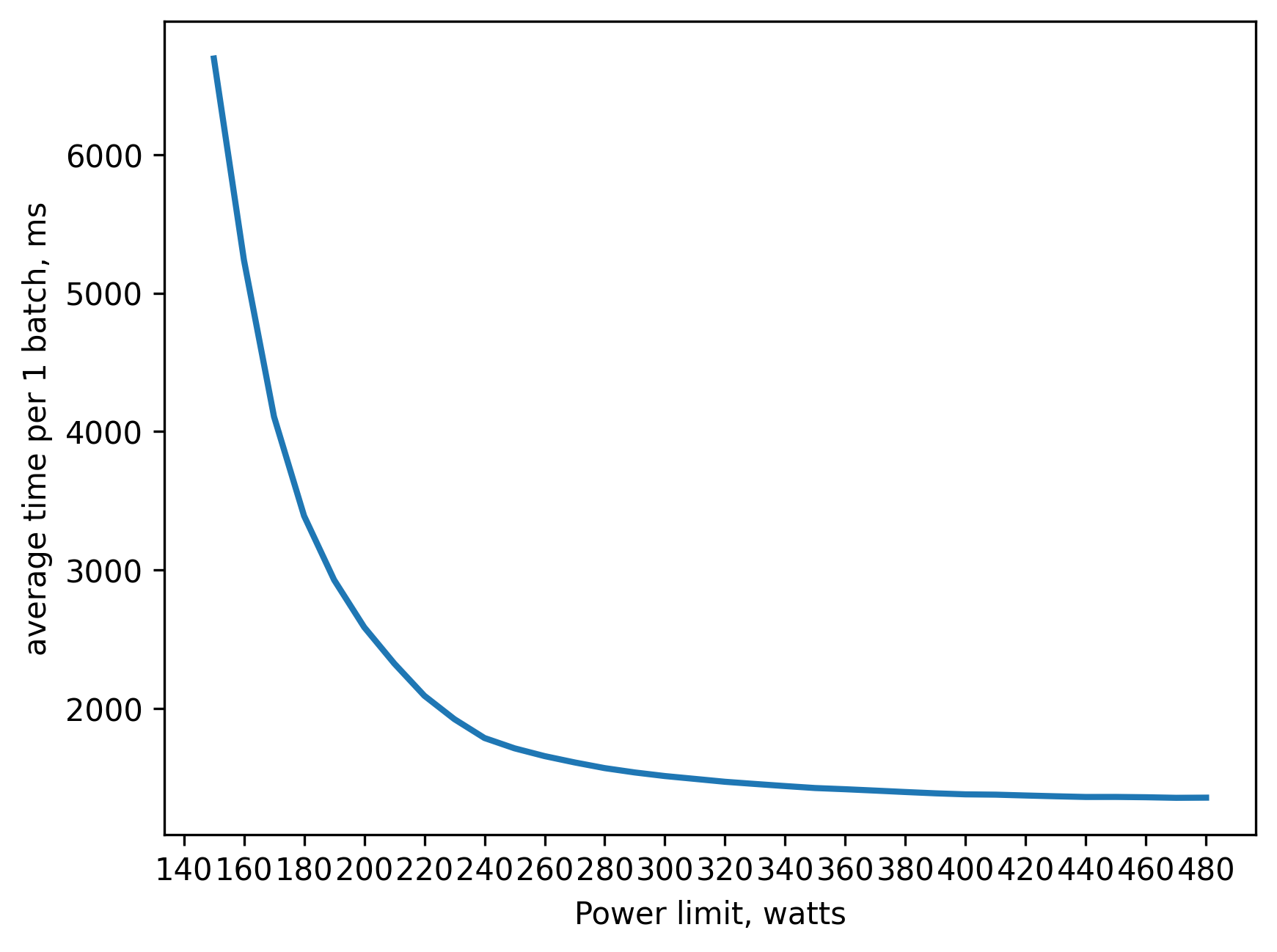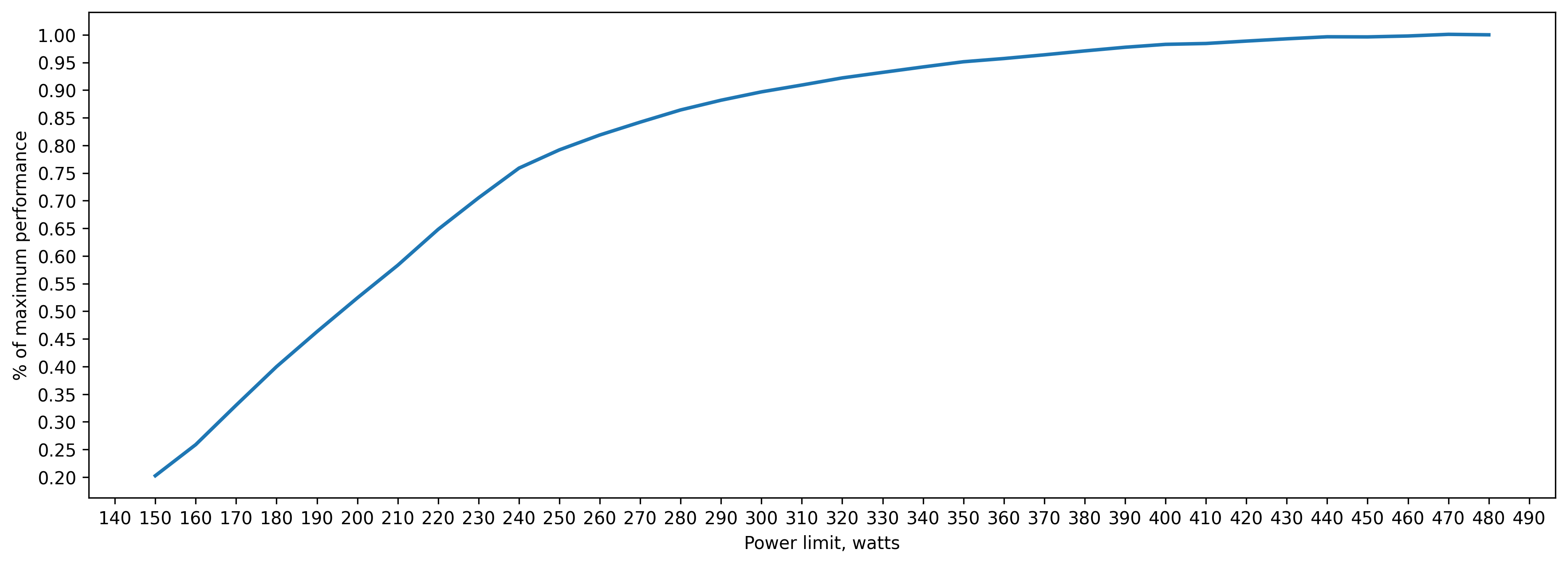260 watts => 80 percent of peak performance(480 watts)
310 watts => 90 percent
360 watts => 95 percent

# fp16 (.half()) inference batch_size=2048 approx. 11GB of VRAM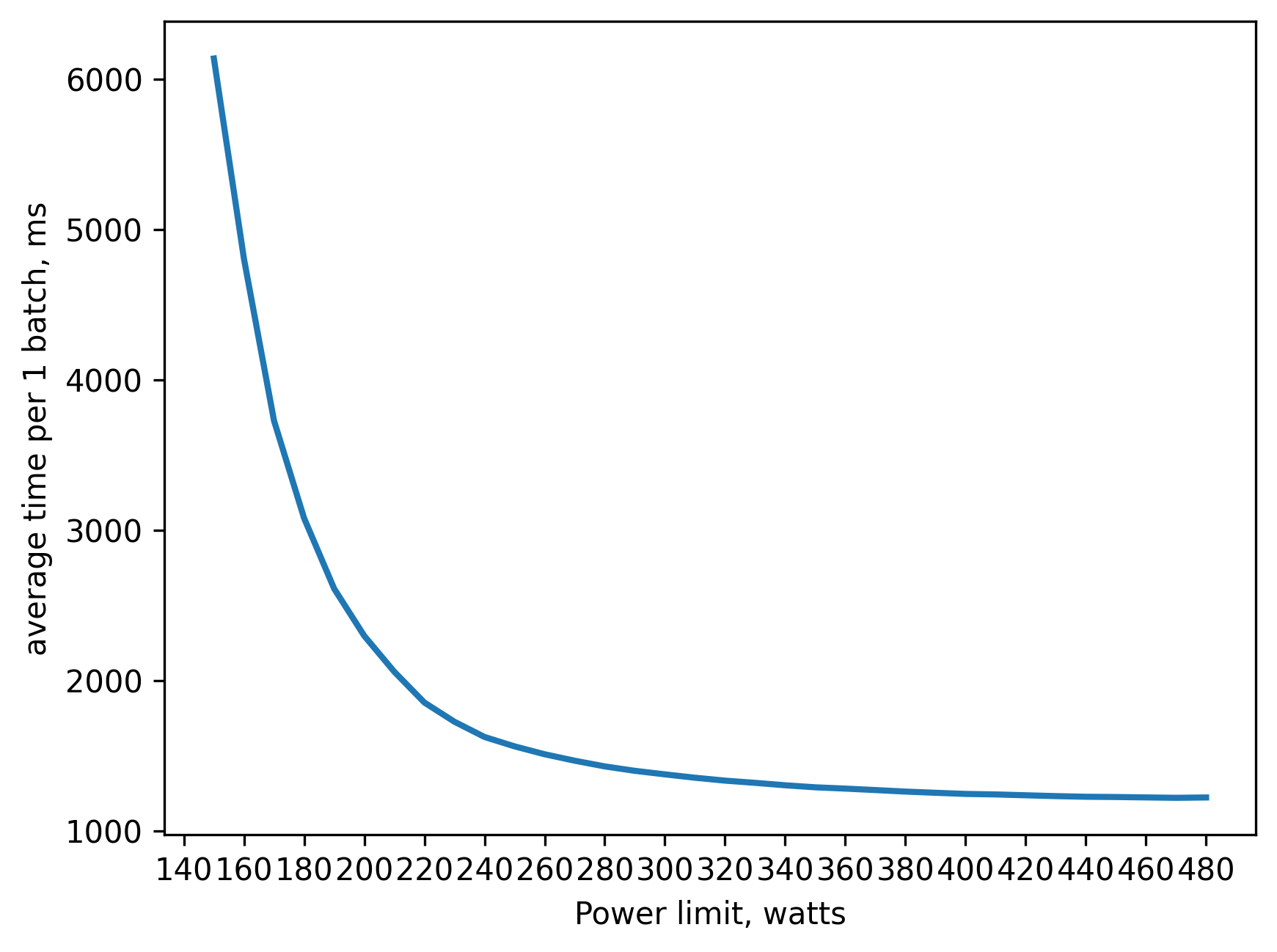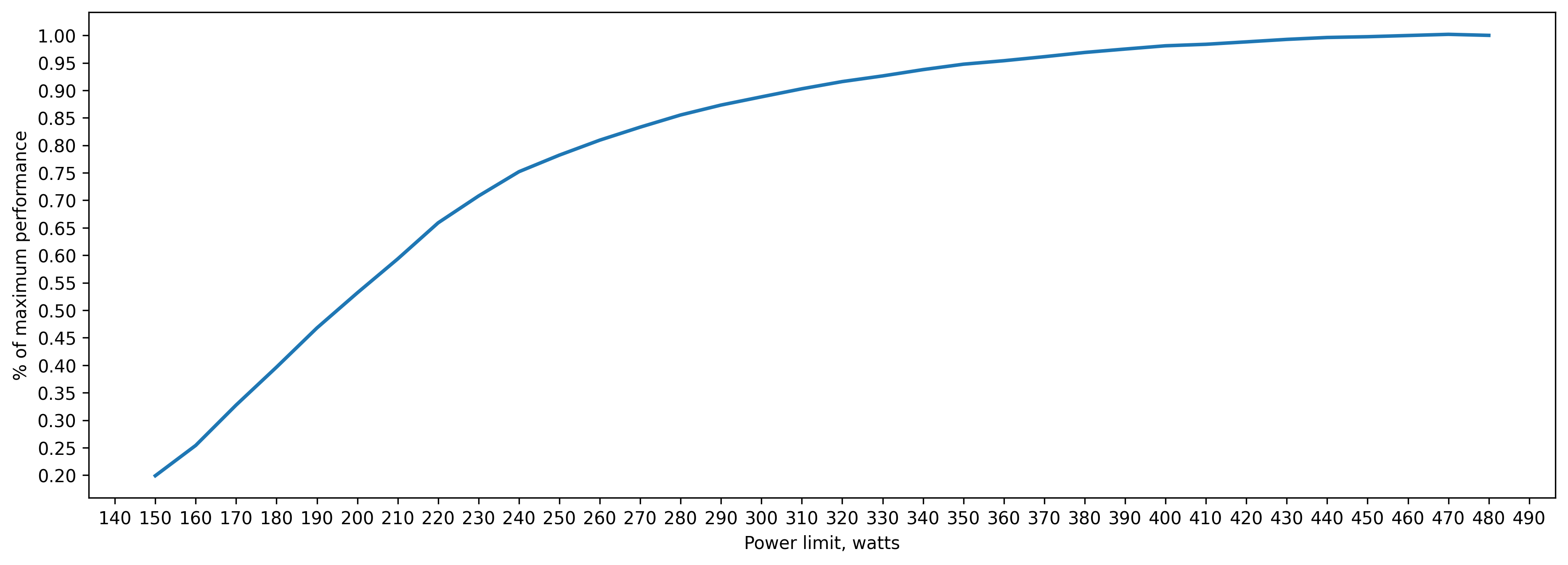260 watts => 80 percent of peak performance(480 watts)
310 watts => 90 percent
360 watts => 95 percent

# TensorRT

We can use TensorRT framework to further optimize our inference. In the next experiments we convert our code to onnx, optimize it, convert onnx to TensorRT and then convert TensorRT model to pytorch jit. At the moment converting pytorch model straight to TensorRT is not working for vision transformer, more details in this issue.

# TensorRT fp16 vs pytorch fp16 (.half()) batch_size=512

Batch size is 512, because I don't have enough VRAM to convert model with bigger batch size to TensorRT.

.half()
260 watts => 82 percent of peak performance(480 watts)
310 watts => 90 percent
360 watts => 95 percent

TensorRT fp16
290 watts => 81 percent of peak performance(480 watts)
340 watts => 90 percent
390 watts => 95 percent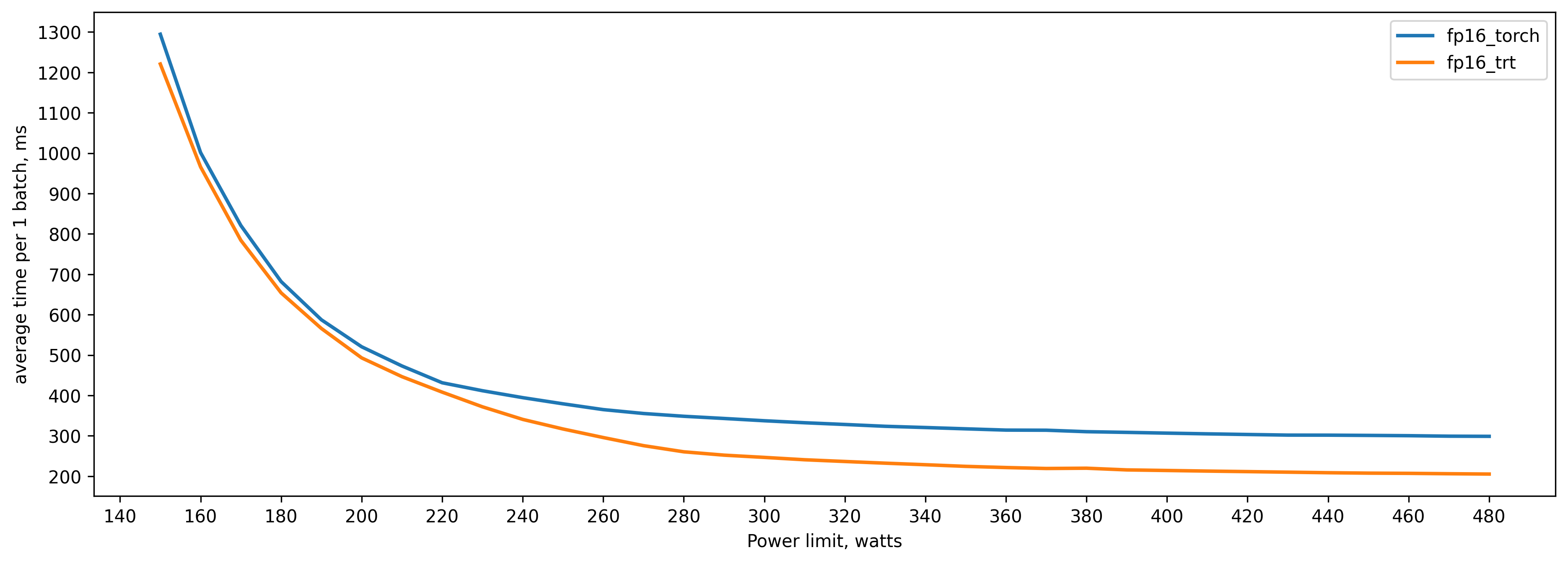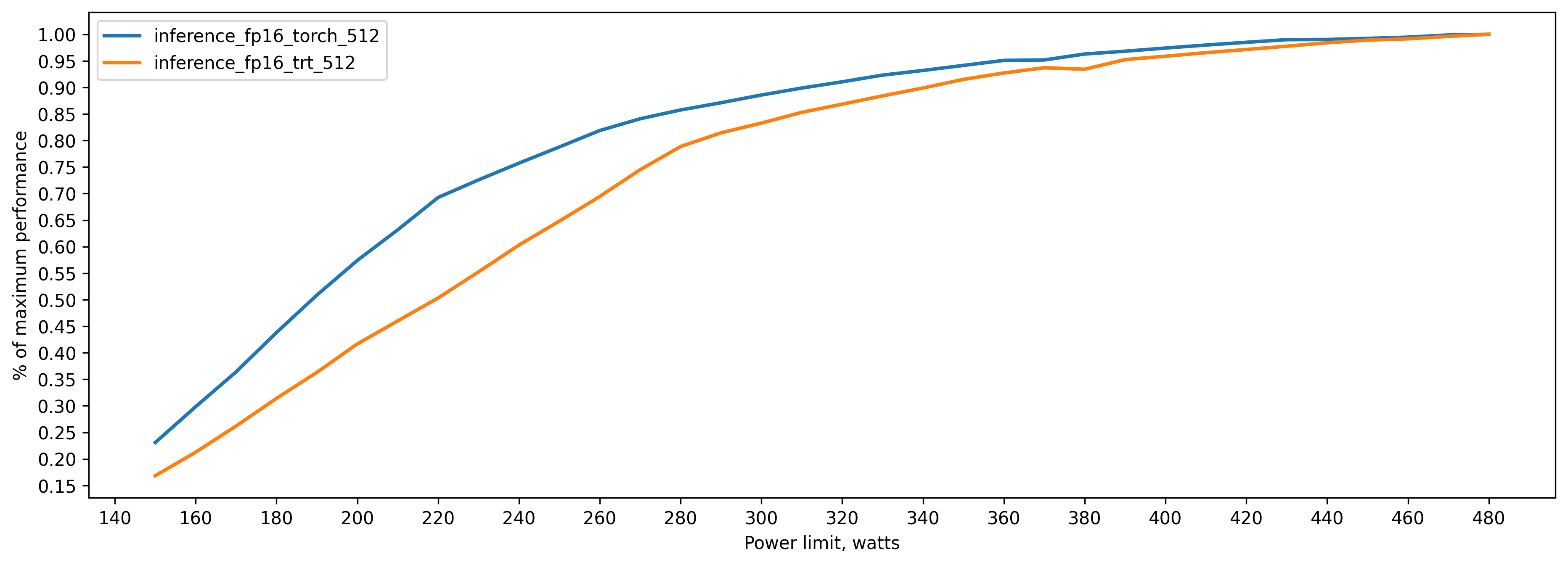Here 100 percent is the maximum performance of TensorRT model: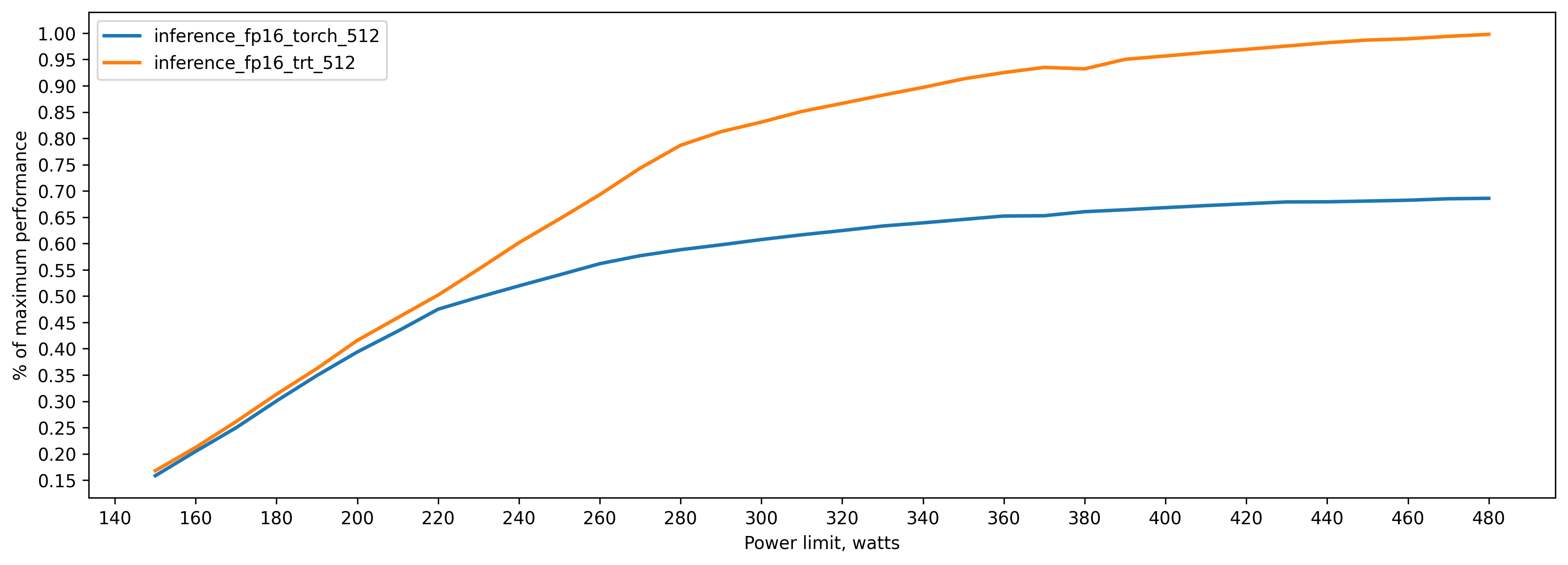# Some more charts

Here 100 percent is the maximum performance of each of the models: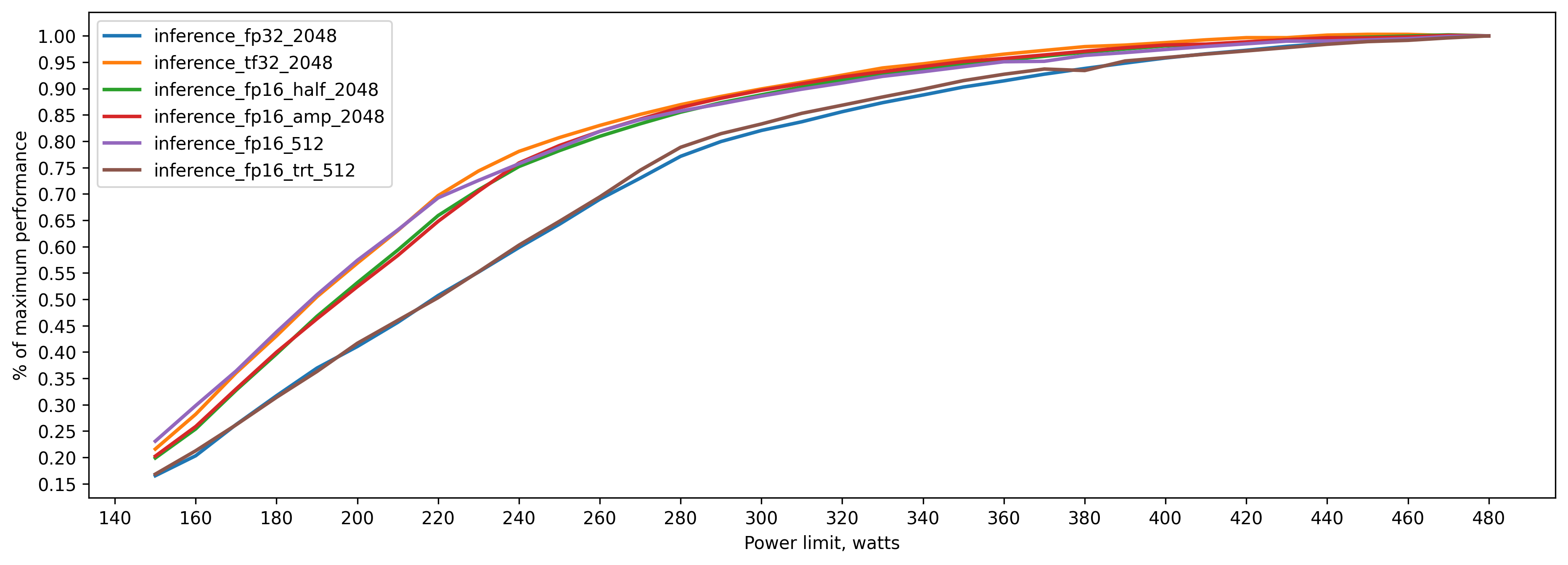Here 100 percent is the maximum performance of fp16 .half() model: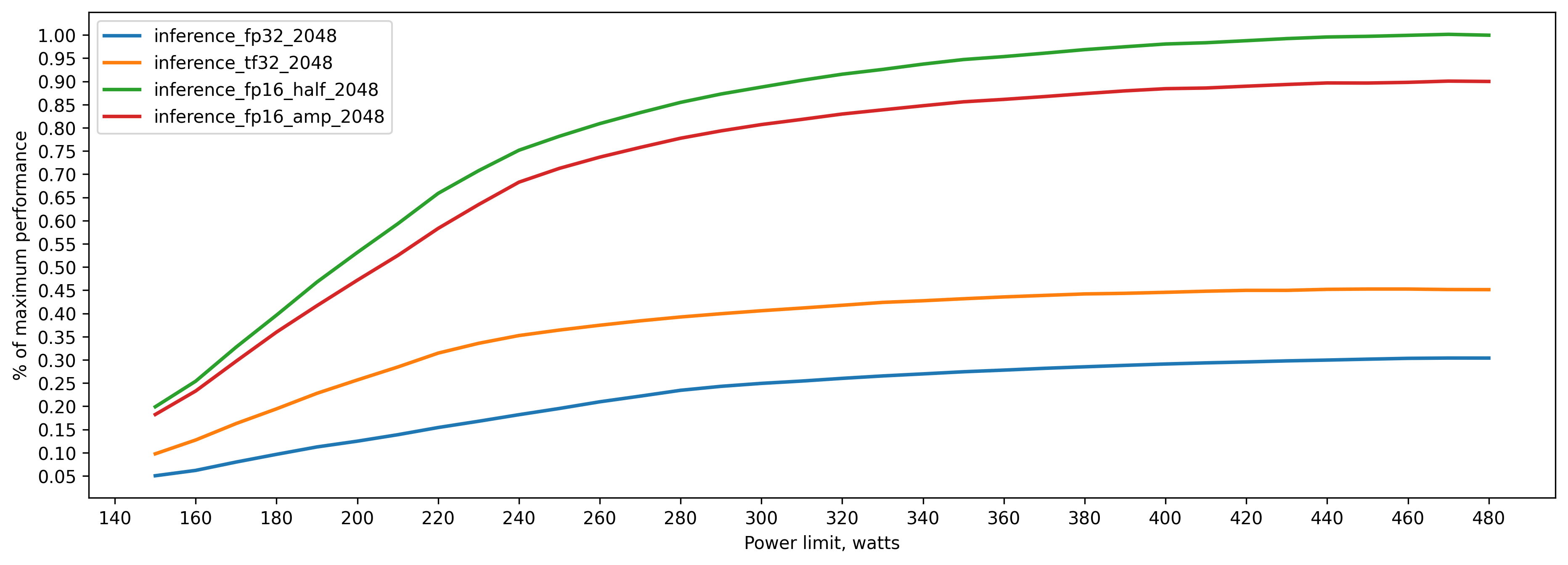Train relative performance.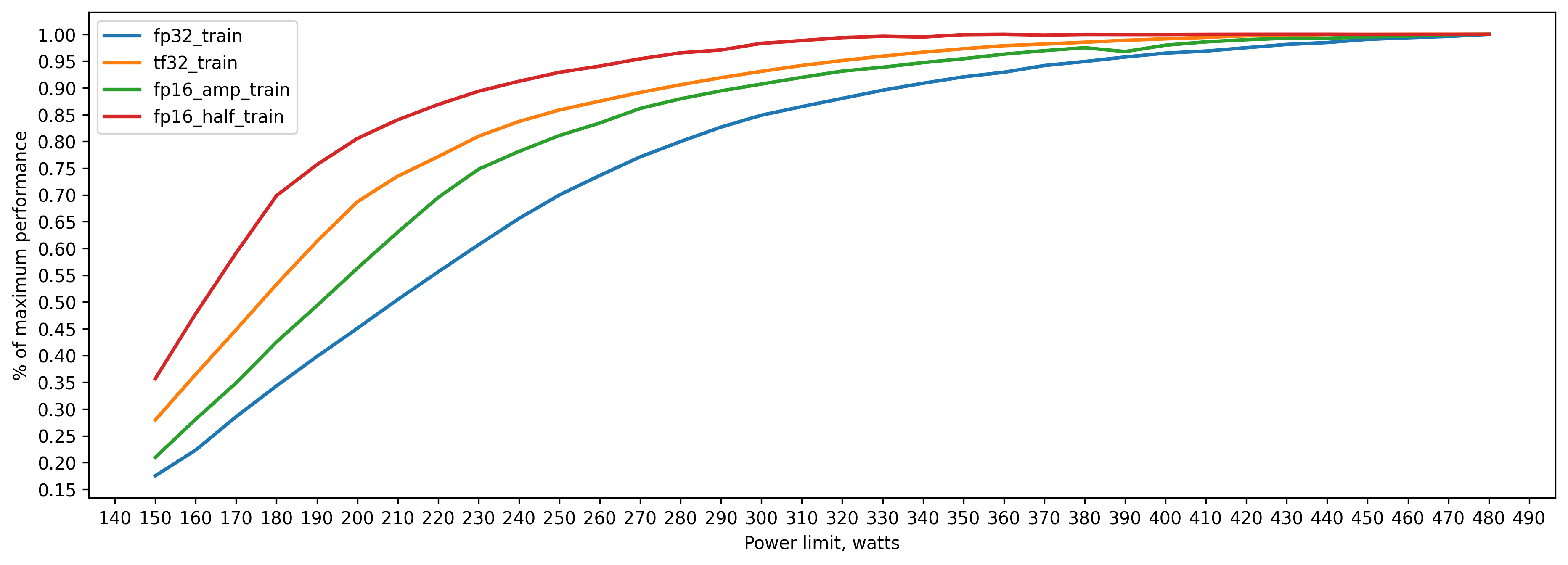Here 100 percent is the maximum performance of fp16 .half() model: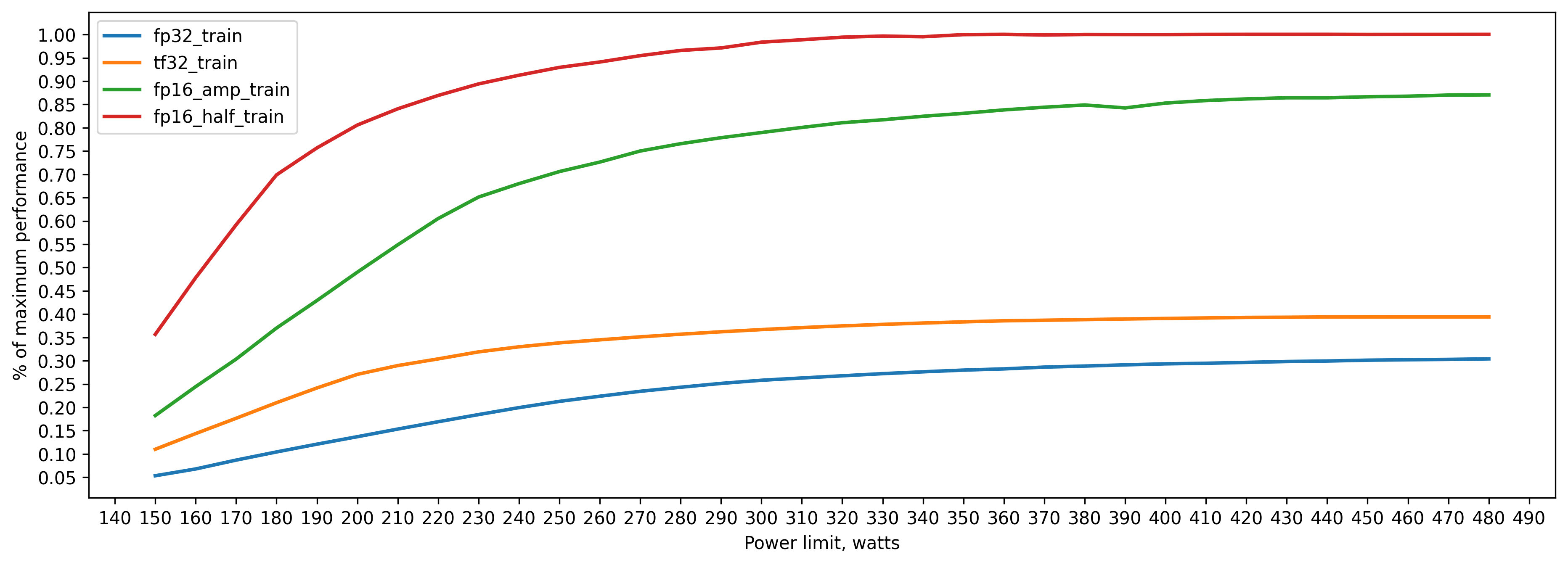# Conclusion

If you want your GPU to overheat less:

• use lower precision. Not only it's faster, but the performance/power curve saturates faster meaning that you can lower power limit without sacrificing much of performance
• 330-360 watts is a good range for the power limit. It might not seem significantly different from the stock limit, but it can make your GPU run a bit cooler (3-5 degrees Celsius) and reduce fan RPM.

Code for all the experiments is available on Github.